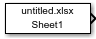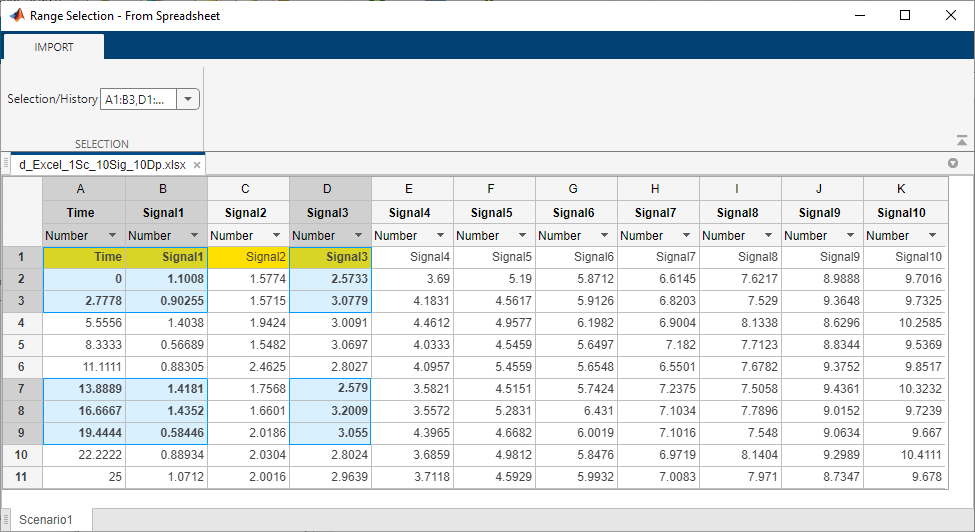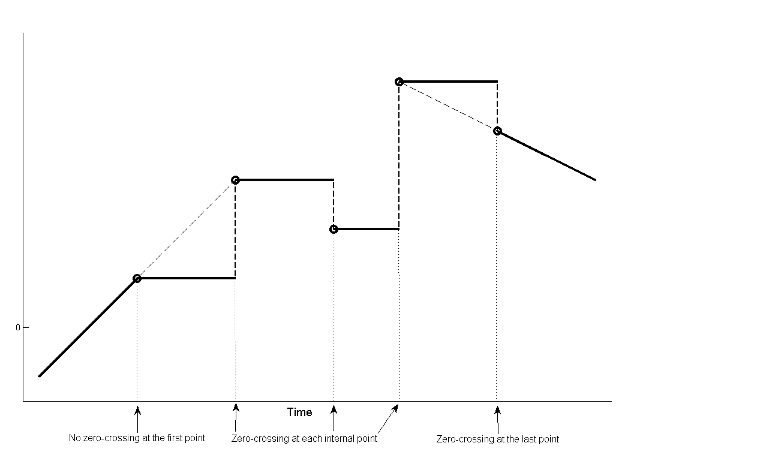• Library:

•## Description

The From Spreadsheet block reads data from Microsoft® Excel® (all platforms) or CSV (MicrosoftWindows® platform with Microsoft Office installed only) spreadsheets and outputs the data as a signal. The From Spreadsheet block does not support Microsoft Excel spreadsheet charts.

The From Spreadsheet icon displays the spreadsheet file name and sheet name specified in the block File name and Sheet name parameters.

### Storage Formats

• The From Spreadsheet block interprets the first row as a signal name. If you do not specify a signal name, the From Spreadsheet block assigns a default one with the format `Signal #`, where `#` increments with each additional unnamed signal.

• The From Spreadsheet block interprets the first column as time. In this column, the time values must monotonically increase.

• The From Spreadsheet block interprets the remaining columns as signals.

This example shows an acceptably formatted Microsoft Excel spreadsheet. The first column is Time and the first row contains signal names. Each worksheet contains a signal group.For CSV text files (Microsoft platform with Microsoft Office installed only):

• The From Spreadsheet block interprets the first column as time. In this column, the time values must increase.

• The From Spreadsheet block interprets the remaining columns as signals.

• Each column must have the same number of entries.

• The From Spreadsheet block interprets each file as one signal group.

This example shows an acceptably formatted CSV file. The contents represent one signal group.

```0,0,0,5,0 1,0,1,5,0 2,0,1,5,0 3,0,1,5,0 4,5,1,5,0 5,5,1,5,0 6,5,1,5,0 7,0,1,5,0 8,0,1,5,1 9,0,1,5,1 10,0,1,5,0 ```

### Block Behavior During Simulation

The Sample time parameter specifies the sample time that the From Spreadsheet block uses to read data from the spreadsheet. For details, see Parameters. The time stamps in the file must be monotonically nondecreasing.

For each simulation time hit for which the spreadsheet contains no matching time stamp, Simulink® software interpolates or extrapolates to obtain the needed data using the selected method. For details, see Simulation Time Hits That Have No Corresponding Spreadsheet Time Stamps.

### Simulation Time Hits That Have No Corresponding Spreadsheet Time Stamps

If the simulation time hit does not have a corresponding spreadsheet time stamp, the From Spreadsheet block output depends on:

• Whether the simulation time hit occurs before the first time stamp, within the range of time stamps, or after the last time stamp

• The interpolation or extrapolation methods that you select

• The data type of the spreadsheet data

For details about interpolation and extrapolation options, see the descriptions of these parameters:

Sometimes the spreadsheet includes two or more data values that have the same time stamp. In such cases, the From Spreadsheet block action depends on when the simulation time hit occurs, relative to the duplicate time stamps in the spreadsheet.

For example, suppose that the spreadsheet contains this data. Three data values have a time stamp value of `2`.

```time stamps: 0 1 2 2 2 3 4 data values: 2 3 6 4 9 1 5 ```

The table describes the From Spreadsheet block output.

Simulation Time, Relative to Duplicate Time Stamp Values in SpreadsheetFrom Spreadsheet Block Action
Before the duplicate time stamps

Performs the same actions as when the time stamps are distinct, using the first of the duplicate time stamp values as the basis for interpolation. (In this example, the time stamp value is `6`.)

At or after the duplicate time stamps

Performs the same actions as when the times stamps are distinct, using the last of the duplicate time stamp values as the basis for interpolation. (In this example, that time stamp value is `9`.)

## Ports

### Output

expand all

Incremental data from the specified spreadsheet.

The Sample time parameter specifies the sample time that the From Spreadsheet block uses to read data from the spreadsheet. For details, see Parameters. The time stamps in the file must be monotonically nondecreasing.

For each simulation time hit for which the spreadsheet contains no matching time stamp, Simulink software interpolates or extrapolates to obtain the needed data using the selected method. For details, see Simulation Time Hits That Have No Corresponding Spreadsheet Time Stamps.

The From Spreadsheet block accepts data type specifications at a block level. If you want to specify different data types for each signal, consider selecting Output Data Type > Inherit: Auto. This option resolves back signal data types using back propagation. For example, assume that there are two signals in the From Spreadsheet block, In1 and In2, which the block sends to ports that have int8 and Boolean data types. With back propagation, the block recasts In1 as int8 and In2 as Boolean.

Data Types: `single` | `double` | `int8` | `int16` | `int32` | `int64` | `uint8` | `uint16` | `uint32` | `uint64` | `Boolean` | `fixed point` | `enumerated`

## Parameters

expand all

Enter full path and file name of a spreadsheet file, including the file extension. If you do not include a file extension, the block assumes `.xlsx` as the extension.

This block supports non-English full paths and file names only on Microsoft platforms.

#### Programmatic Use

 Block Parameter: `FileName` Type: character vector Value: full path and file name Default: `'untitled.xlsx'`

Enter the name of the sheet in the spreadsheet. You can type the sheet name in this edit box or select the sheet name after you open the sheet.

If your spreadsheet is the CSV format, the block populates this parameter with the name of the CSV file without the extension. Do not change this value.

To open the sheet, click. In the sheet, you can select the range of data by dragging over the desired range of values.Alternatively, you can select the range of data by specifying the range of values in the Range parameter.

#### Programmatic Use

 Block Parameter: `SheetName` Type: character vector Value: Sheet name Default: `'Sheet1'`

To specify the range, use the format `column:row`, with multiple specifications separated by commas. For example, `A1:B3,D1:D3,A7:B9,D7:D9`. If unspecified, or empty, the block automatically detects the used range, which is all the data in the sheet.If the selections overlap, the block resolves the selection information as appropriate. For example, if you specify multiple ranges that overlap, such as `A1:B4,B1:E7`, the block resolves the selection to A1 to E7, inclusive.

An alternate to using the Range parameter is to open the sheet, by clicking. In the sheet, you can select the range of data by dragging over the desired range of values.

#### Programmatic Use

 Block Parameter: `Range` Type: character vector Value: Cell range Default: `''`

The data type for the From Spreadsheet block output. The From Spreadsheet block accepts spreadsheets that contain many data types. However, the block reads the spreadsheet data type as doubles. It then outputs the data type according to the value of Output data type.

If you want to specify different data types for each signal, consider selecting Output Data Type > Inherit: auto. This option resolves back signal data types using back propagation. For example, assume that there are two signals in the From Spreadsheet block, In1 and In2, which the block sends to ports that have int8 and Boolean data types. With back propagation, the block recasts In1 as int8 and In2 as boolean.

To allow the block to cast the output data type to match that of the receiving block, use `Inherit: auto`.

#### Programmatic Use

 Block Parameter: `OutDataTypeStr` Type: character vector Values: ```'Inherit: auto' | 'double' | 'single' | 'int8' | 'uint8' | 'int16' | 'uint16' | 'int32' | 'uint32' | 'int64' | 'uint64' | 'boolean' | 'fixdt(1,16,0)' | 'fixdt(1,16,2^0,0)' | 'Enum: ' | ''``` Default: `'Inherit: auto'`

Select how the block should treat the first column of the spreadsheet:

• `Time` — Treat first column as time.

• `Data` — Treat first column as data.

#### Dependencies

When you select `Data`, the block disables:

• Data extrapolation before first data point

• Data interpolation within time range

• Data extrapolation after last data point

And enables:

• Output after last data point

#### Programmatic Use

 Block Parameter: `TreatFirstColumnAs` Type: character vector Value: `'Time' | 'Data'` Default: `'Time'`

The sample period and offset.

• You specify for the From Spreadsheet block

• The From Spreadsheet block inherits from the blocks into which the From Spreadsheet block feeds data

The default is `0`, which specifies a continuous sample time. The spreadsheet is read at the base (fastest) rate of the model. For details, see Specify Sample Time.

#### Programmatic Use

 Parameter: `SampleTime` Type: character vector Values: scalar | vector Default: `'0'`

Extrapolation method that Simulink uses for a simulation time hit that is before the first time stamp in the spreadsheet. Choose one of these extrapolation methods.

MethodDescription
`Linear extrapolation`

(Default)

If the spreadsheet contains only one sample, the From Spreadsheet block outputs the corresponding data value.

If the spreadsheet contains more than one sample, the From Spreadsheet block linearly extrapolates using the first two samples:

• For `double` data, linearly extrapolates the value using the first two samples

• For `Boolean` data, outputs the first data value

• For a built-in data type other than `double` or `Boolean`:

• Upcasts the data to `double`

• Performs linear extrapolation (as described above for `double` data)

• Downcasts the extrapolated data value to the original data type

You cannot use the ```Linear extrapolation``` option with enumerated (`enum`) data.

`Hold first value`

Uses the first data value in the file

`Ground value`

Uses a value that depends on the data type of spreadsheet sample data values:

• Fixed-point data types — Uses the ground value

• Numeric types other than fixed–point — Uses `0`

• `Boolean` — Uses `false`

• Enumerated data types — Uses default value

#### Dependencies

To enable this parameter, set Treat first column as to `Time`.

#### Programmatic Use

 Parameter: `ExtrapolationBeforeFirstDataPoint` Type: character vector Values: ```'Linear extrapolation' | 'Hold first value' | 'Ground value'``` Default: `'Linear extrapolation'`

The interpolation method that Simulink uses for a simulation time hit between two time stamps in the spreadsheet. Choose one of the following interpolation methods.

MethodDescription
`Linear interpolation`

(Default)

• For `double` data, linearly interpolates the value using the two corresponding samples

• For Boolean data, if the simulation time is between two spreadsheet data points with different values, the From Spreadsheet block positions the value transition halfway between the spreadsheet data points. For example, if the spreadsheet data transitions from true to false, the From Spreadsheet data provides an output value of true for simulation times in the first half of the interval between the spreadsheet data points. It provides an output value of false for simulation times in the latter half of the interval.

• For a built-in data type other than `double` or `Boolean`:

• Upcasts the data to `double`

• Performs linear interpolation (as described above for `double` data)

• Downcasts the interpolated value to the original data type

You cannot use the ```Linear interpolation``` option with enumerated (`enum`) data.

`Zero order hold`

Uses the data from the first of the two samples

#### Dependencies

To enable this parameter, set Treat first column as to `Time`.

#### Programmatic Use

 Parameter: `InterpolationWithinTimeRange` Type: character vector Values: `'Linear interpolation' | 'Zero order hold'` Default: `'Linear interpolation'`

The extrapolation method that Simulink uses for a simulation time hit that is after the last time stamp in the spreadsheet. Choose one of the following extrapolation methods.

MethodDescription
`Linear extrapolation`

(Default)

If the spreadsheet contains only one sample, the From Spreadsheet block outputs the corresponding data value.

If the spreadsheet contains more than one sample, the From Spreadsheet block linearly extrapolates using data values of the last two samples:

• For `double` data, extrapolates the value using the last two samples

• For `Boolean` data, outputs the last data value

• For a built-in data type other than `double` or `Boolean`:

• Upcasts the data to `double`.

• Performs linear extrapolation (as described above for `double` data).

• Downcasts the extrapolated value to the original data type.

You cannot use the ```Linear extrapolation``` option with enumerated (`enum`) data.

`Hold last value`

Uses the last data value in the file

`Ground value`

Uses a value that depends on the data type of spreadsheet sample data values:

• Fixed-point data types — Uses the ground value

• Numeric types other than fixed–point — uses `0`

• `Boolean` — Uses `false`

• Enumerated data types — Uses default value

#### Dependencies

To enable this parameter, set Treat first column as to `Time`.

#### Programmatic Use

 Parameter: `ExtrapolationAfterLastDataPoint` Type: character vector Values: ```'Linear extrapolation' | 'Hold last value' | 'Ground value'``` Default: `'Linear extrapolation'`

Select action after last data point:

• `Repeating sequence` — Repeat the sequence by reading the data from the first row of the range specified in Range

• `Hold final value` — Output the last defined value for the remainder of the simulation.

• `Ground value` — Output a ground value depending on the data type value specified in Output data type.

#### Dependencies

To enable this parameter, set Treat first column as to `Data`.

#### Programmatic Use

 Parameter: `OutputAfterLastPoint` Type: character vector Values: ```'Repeating sequence' | 'Hold final value' | 'Ground value'``` Default: `'Repeating sequence'`

Select to enable zero-crossing detection.

The Zero-Crossing Detection parameter applies only if the Sample time parameter is set to `0` (continuous).

Simulink uses a technique known as zero-crossing detection to locate accurately a discontinuity, without resorting to excessively small time steps. In this context, zero-crossing is used to represent discontinuities.

For the From Spreadsheet block, zero-crossing detection can only occur at time stamps in the file. Simulink examines only the time stamps, not the data values.

If the input array contains duplicate time stamps (more than one entry with the same time stamp), Simulink detects a zero crossing at that time stamp. For example, suppose that the input array has this data.

```time: 0 1 2 2 3 signal: 2 3 4 5 6 ```

At time 2, there is a zero crossing from the input signal discontinuity.

For data with nonduplicate time stamps, zero-crossing detection depends on the settings of the following parameters:

• Data extrapolation before first data point

• Data interpolation within time range

• Data extrapolation after last data point

The block applies the following rules when determining when:

• Zero-crossing occurs for the first time stamp

• For time stamps between the first and last time stamp

• For the last time stamp

Time StampWhen Zero-Crossing Detection Occurs

First

Data extrapolation before first data point is set to ```Ground value```.

Between first and last

Data interpolation within time range is set to ```Zero-order hold```.

Last

One or both of these settings occur:

• Data extrapolation after last data point is set to ```Ground value```.

• Data interpolation within time range is set to ```Zero-order hold```.

The following figure illustrates zero-crossing detection for data accessed by a From Spreadsheet block that has these settings:

• Data extrapolation before first data point`Linear extrapolation`

• Data interpolation within time range (for internal points) — ```Zero order hold```

• Data extrapolation after last data point`Linear extrapolation`The following figure is another illustration of zero-crossing detection for data accessed by a From Spreadsheet block. The block has these settings for the time stamps (points):

• Data extrapolation before first data point`Hold first value`

• Data interpolation within time range`Zero order hold`

• Data extrapolation after last data point`Hold last value`#### Programmatic Use

 Block Parameter: `ZeroCross` Type: character vector Values: `'off'` | `'on'` Default: `'off'`

## Block Characteristics

 Data Types `Boolean` | `double` | `enumerated` | `fixed point[a]` | `integer` | `single` Direct Feedthrough `no` Multidimensional Signals `no` Variable-Size Signals `no` Zero-Crossing Detection `yes` [a] Supports up to 32-bit fixed-point data types.

## Algorithms

expand all

When the From Spreadsheet block reads data from a spreadsheet, it reads all signals with double precision and then casts them to the data type specified in the Output data type parameter for the output signal. During casting, the block uses rounding mode and saturation on integer overflow as follows.